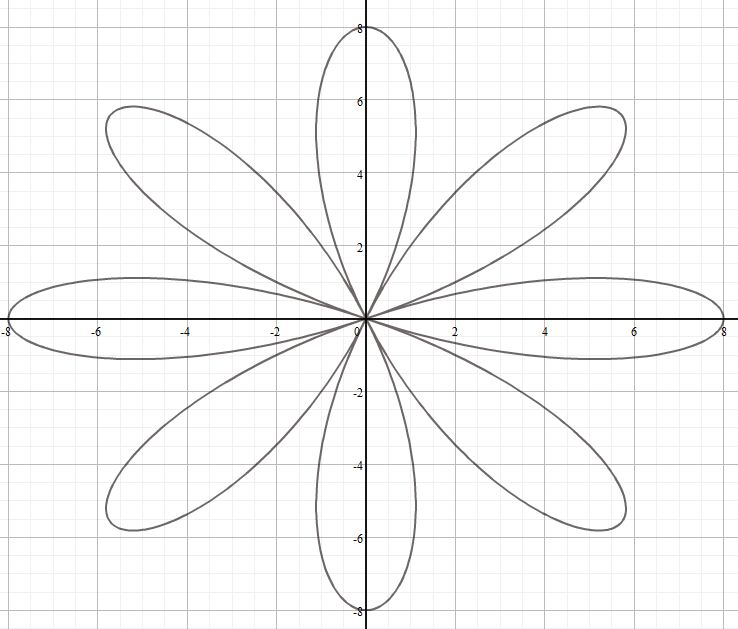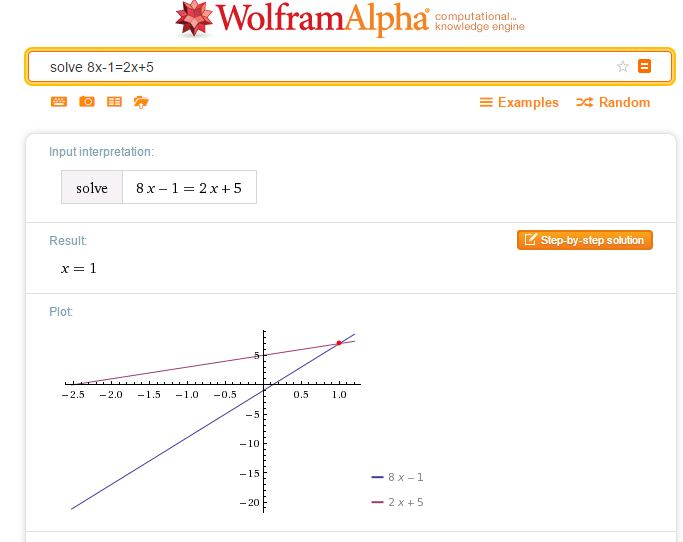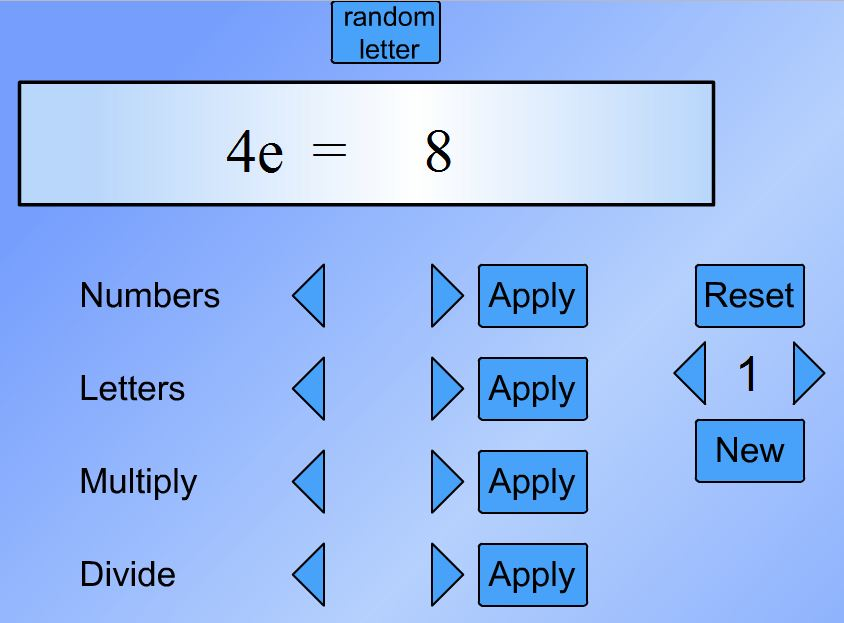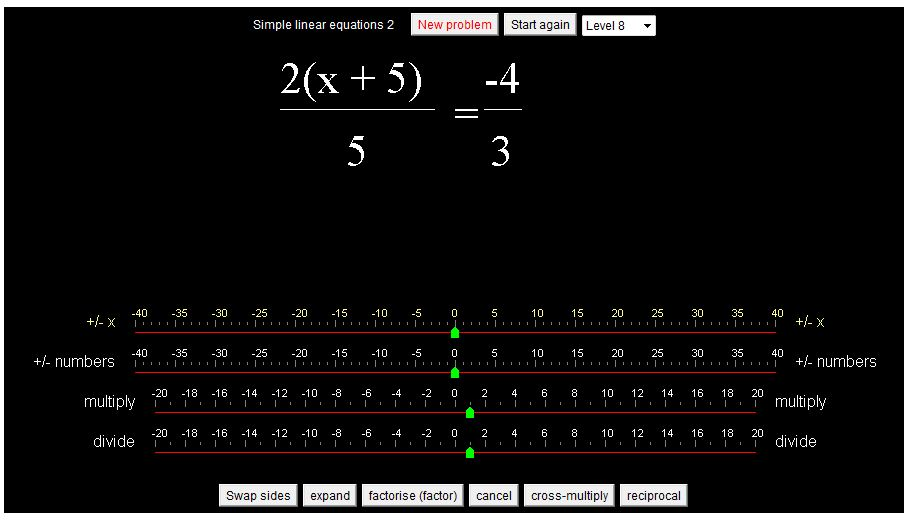# For Students….

With older students in mind including those about to enter higher education, there are many free resources available to support students in their study of Mathematics.
Note that a new blog aimed at students is now available.

There are many sources of excellent notes online. See this Evernote shared notebook: Mathematics notes for useful links. (You do not need an Evernote account to view the notebook). Some of these sites include videos, for an extensive collection of videos try Khan Academy.
Alternatively, use the Livebinders version of Mathematics Notes.

On the subject of Evernote – it is a valuable tool for any student or teacher.
Other tools which may be of interest are on Digital Tools for Students.

Students going on to study Mathematics degrees have a look at Kevin Houston’s ‘How not to get a good mathematics degree‘ and ‘How to get a good mathematics degree‘. He also has provided a pdf file you can download: 10 Ways to Think Like a Mathematician. Kevin Houston works at the University of Leeds.

I would advise students of any subject to learn to use WolframAlpha. Suppose you wish to do some revision on techniques for differentiation for example – ask WolframAlpha to differentiate x2sinx, and the derivative will be returned with possible working available if  ‘Show steps’ is selected. (Further Calculus examples). WolframAlpha provides an excellent way to check your work.

WolframAlpha will plot graphs, there are many other free and excellent online graphing tools, see the Desmos Graphing Calculator for example and many other online graphing tools. (See this page for polar curve plotters).Thinking longer term – have a look at the excellent Maths Careers site.

# Words with WolframAlpha

WolframAlpha can be used for vocabulary, for example try typing ‘plus’ into WolframAlpha.Note that WolframAlpha has assumed plus is a character but you can choose to refer to a mathematical definition or a word (or an internet architecture topic). Choosing word will not only give definitions but a wealth of other information that you would not find in a dictionary such as the first known use of the word, frequency of use, rhyming words and much more!

Other sites of interest for Mathematics vocabulary have been mentioned in this Mathematics Reference post. If you are interested in the first know use of mathematical words then try Jeff Miller’s ‘Earliest Known Uses of  Some of the Words of Mathematics‘.

# Solving Equations

A collection of resources to use to demonstrate and practise solving equations.A simple way to check a solution to an equation of any type is to simply enter your equation as a WolframAlpha query. Note that WolframAlpha includes a graphical illustration; it is so important for students to understand how equations may be solved graphically; I always illustrate graphical solutions when we are working with Algebra to help students make these links. Desmos of course, is ideal here.The following resources allow practice in choosing the correct operations to reach a solution.

Centre for Innovation in Mathematics Teaching
CIMT
have tutorials on equations: Linear Equations 1, Linear Equations 2, and Linear Equations with Brackets in their Interactive ResourcesChoose the type of equation you require then the sequence of operations required to solve the equation.
Select Do it after each operation, for example -32 Do it were the keys selected to start the above problem.

The next sites work in a similar way to the subtangent resource.

Flashy MathsSolving Equations (select the swf file to play online)There are 4 levels to choose from: the level 1 equations are of the type ax = b,
level 2, x±c = d, level 3, ex±f = g and level 4, hx±i = hx±j = k.
Choose the series of operations you require to solve the equation selecting apply at each stage to see the result of your chosen operation.
Choose numbers to add and subtract integers and letters to add and subtract variables.

MathsNetEquation Buster
Playing at level 5 introduces equations with fractions.From the National Library of Virtual Manipulatives try Algebra Balance Scales and Algebra Balance Scales – Negatives.
Note that these manipulatives allow you to create a problem of your own (so long as it has an integer solution) as well as solving problems given.From WaldoMaths, try this applet on solving simple linear equations and this on equations requiring more complex manipulation.
Each applet has several levels.Unlike the other resources this allows the user to enter the equation resulting from the chosen operation.
The solution is checked at each stage.
As well as solving the given problems (20 problems) the user can select ‘Make an equation yourself’ to enter any equation then solve that equation.
(Unlike the NLVM resource above the x can be any real number).

The following video shows the keystrokes required to enter and solve an equation.